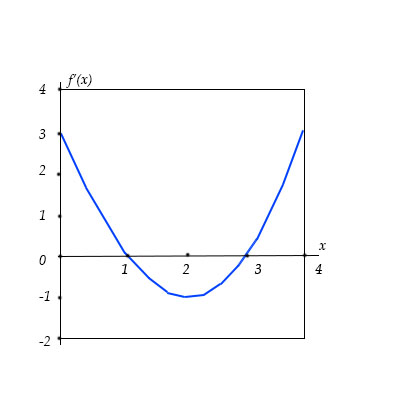# Use the graph of y=f'(x) shown below to describe the concavity in the graph of y=f(x) on the...

## Question:

Use the graph of {eq}y=f'(x) {/eq} shown below to describe the concavity in the graph of {eq}y=f(x) {/eq} on the interval {eq}[0,4]. {/eq}## Concavity:

When we have the graph of the first derivative of the function: y=f(x) given in the question, then to find the concavity of the inflection point, we will find the second-order derivative.

Now to find the concavity, we will find the second-order derivative and will see where the functions' second derivative is positive and negative.

For the positive second-order derivative, we will have the concave upward graph of the function y=f(x).

And negative second orde derivative means the concave downward graph.

Now we will see that the second-order derivative for the first-order derivative's graph is negative in the interval: (3,2) and thus in this interval, the function y=f(x) is concave downward.

Also, the second-order derivative is positive in the interval (2,4) and hence in this interval, the function is concave upward.